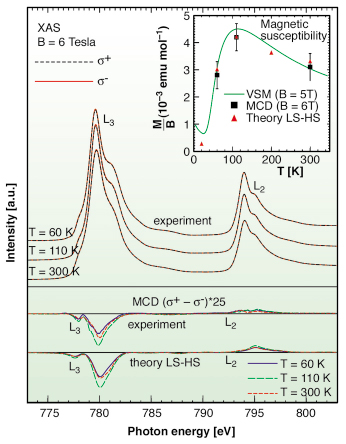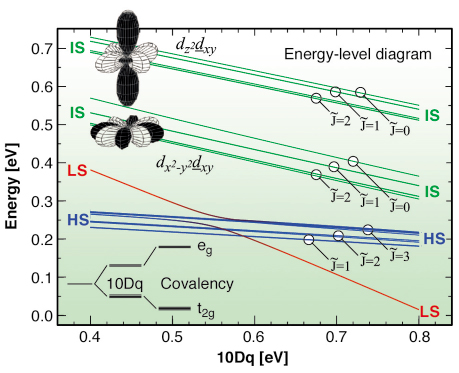LaCoO3 shows a gradual non-magnetic to magnetic transition with temperature, the nature of which has been highly debated over the last few decades. Starting from a low-spin (LS, t2g6eg0, S=0) ground state for the Co3+ ion at low temperatures, either a high-spin (HS, t2g4eg2, S=2) or an intermediate-spin (IS, t2g5eg1, S=1) first excited state becomes populated at elevated temperatures. In the recent past, local density approximation + Hubbard U (LDA+U) band structure calculations predict the IS state to be lower in energy than the HS . Nowadays, this forms the basis of most explanations for the fascinating properties of the recently synthesised layered cobaltate materials, which show giant magnetoresistance and metal-insulator/magnetic transitions with various forms of charge, orbital, and spin ordering. In this work carried out at the ID08 beamline, we examined the validity of this prediction and have arrived at an unexpected conclusion.

We used soft X-ray absorption spectroscopy (XAS) and magnetic circular dichroism (MCD) at the Co L2,3 edge. Figure 113 shows the XAS and MCD spectra taken at 60, 110 and 300 K, i.e. in the paramagnetic phase, using a 6 Tesla magnet. The MCD signal is only in the order of 1%, but can nevertheless be measured very reliably due to the good signal to noise ratio, stability of the beam, and the accurate photon energy referencing. In the figure, the experimental MCD curves are compared to theoretical spectra calculated for a CoO6 cluster. We are able to obtain good agreement for an inhomogeneously mixed LS-HS scenario (see Figure 113), but not for a mixed LS-IS.Fig. 113: Top: experimental Co-L2,3 XAS spectra taken from LaCoO3 at 60, 110, and 300 K using circularly polarised X-rays with the photon spin aligned parallel (+) and antiparallel (-) to the 6 Tesla magnetic field. Middle: experimental MCD spectra. Bottom: theoretical MCD spectra calculated in the LS-HS scenario. Inset: Magnetic susceptibility measured by VSM (solid line), calculated from the cluster (triangles) using the HS population obtained from the XAS spectra and extracted from MCD data (squares).

To check our data, we use the MCD sum-rules to extract the values for the orbital (Lz) and spin (2Sz) contributions to the induced moments. These results normalised to the magnetic field are plotted in the inset of Figure 113. They are in close agreement with the magnetic susceptibility as measured using a vibrating sample magnetometer (VSM).

An important aspect that emerges directly from the MCD experiments is the presence of a very large induced orbital moment: we find that Lz/Sz ~ 1/2. This means first of all that the spin-orbit coupling must be considered in evaluating the energy level diagram, as is done in Figure 114. Second, and crucial for the spin-state issue, this large Lz/Sz ratio allows us to directly verify the LS-HS scenario and falsify the LS-IS. One should realise that a t2g electron has a pseudo orbital momentum of 1, which couples with the spin. For the HS state we find a lowest state that is a triplet, with Lz = 0.6, Sz = 1.3 and a g-factor of 3.2, in good agreement also with ESR experiments. For the IS state, the hole in the t2g shell couples with the electron in the eg shell due to the strong orbital dependent Coulomb interactions leading to a dx2-y2dxy state plus their x, y, z-cyclic permutations (see Figure 114). The eg electron in turn, couples to the lattice and this leads, according to LDA+U , to the formation of an orbital ordered state. This ‘real-space’ state, however, does not carry a large orbital momentum and is therefore not compatible with the values observed in our MCD measurements.Fig. 114: Energy level diagram of a CoO6 cluster as a function of the ionic part of the crystal field splitting 10Dq.

To summarise, we find spectroscopic evidence that the spin-state transition in LaCoO3 can be well described by a LS ground state and a triply degenerate HS excited state, and that at elevated temperatures an inhomogeneous mixed-spin state system is formed. The large orbital momentum revealed by the MCD measurements invalidates existing LS-IS scenarios. Consequently, the spin-state issue for the new class of the layered cobaltates needs to be reinvestigated.

Reference

 M. A. Korotin et al., Phys. Rev. B 54, 5309 (1996).

Principal Publication and Authors

M.W. Haverkort (a), Z. Hu (a), J.C. Cezar (b), T. Burnus (a), H. Hartmann (a), M. Reuther (a), C. Zobel (a), T. Lorenz (a), A. Tanaka (c), N.B. Brookes (b), H.H. Hsieh (d,e), H.-J. Lin (e), C.T. Chen (e), and L.H. Tjeng (a), Phys. Rev. Lett. 97, 176405 (2006).
(a) II. Physikalisches Institut, Universität zu Köln (Germany)
(b) ESRF